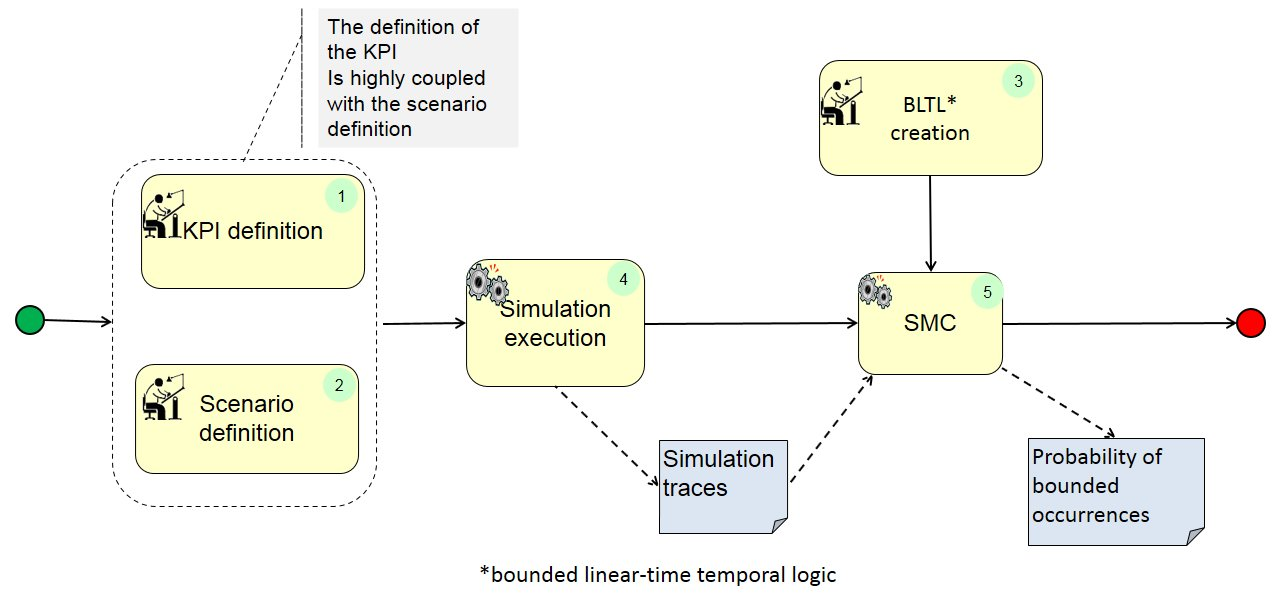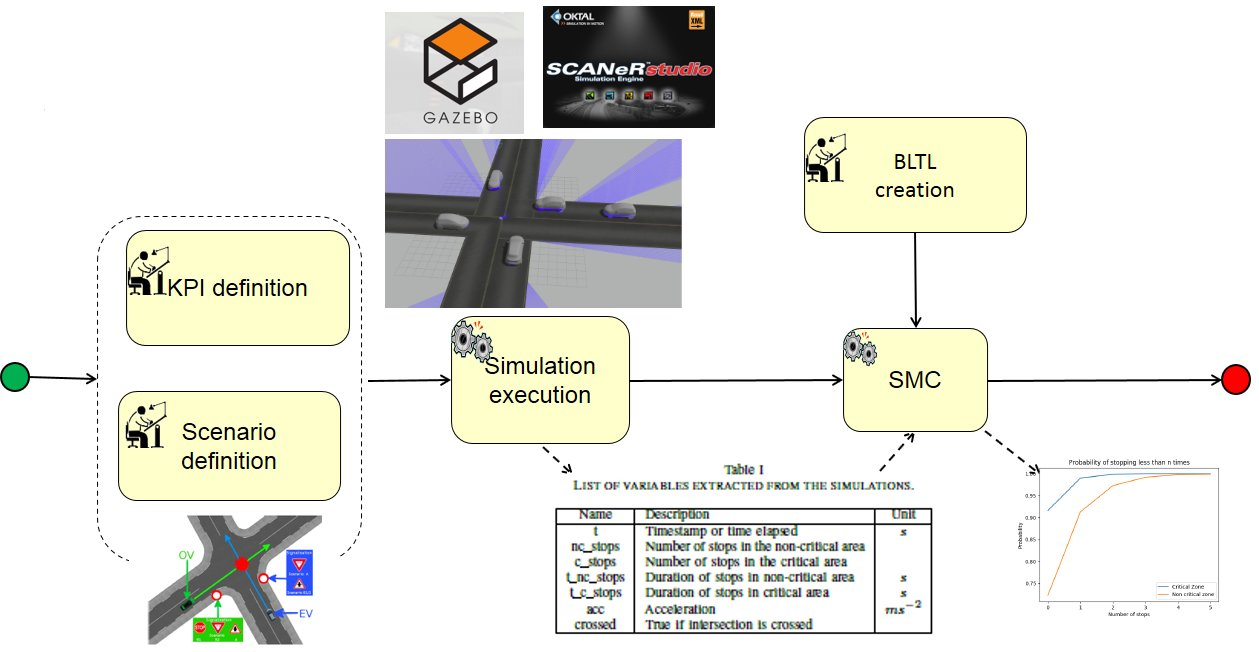# Statistical Model Checking based Validation

#### Meta-Information

Origin: Mathieu Barbier / INRIA, Jean Quilbeuf / INRIA, Alessandro Renzaglia / INRIA, Christian Laugier / INRI

Written: April 2019

Purpose: Application of the statistical model checking to validate probabilistic decision making and perception algorithms.

Context/Pre-Conditions: availability of
– KPI definition, Scenario definition
– Working simulation platform

To consider: The boundary for the analysis must be set by experts.

#### Structure#### Participants and Important Artefacts

Simulation engineer: In charge of the setup of the simulation platform and the generation of traces.

Validation engineer: In charge of defining BLTL statement.

System expert: In charge of defining relevant KPI for the SUT.

Simulation traces: Executions of the system generated according to the probabilities in the model.

Probability of bounded occurrences: The final result is given in terms of probability to meet a certain property expressed as a BLTL formula.

#### Actions/Collaborations

(1.a) The first step is to look at criteria that could be used to judge performances of the ACPS.

(1.b) Then, given the scenario, some KPI must be defined to validate the behavior of the ACPS in the scenario.

(2) Scenario definition:

(2.a) The first task is to defined dynamic and static elements that the ACPS will have to interact with.

(2.b) For each element included in the scenario, find what variability is required for each characteristics of an element (e.g. initial velocity of a vehicle).

(2.c) Then, find criteria that will be used to determine whether the test was successful or not.

(3) BLTL creation: Transform success criteria and KPI into BLTL expressions that can be used by the SMC to estimate the probability of remaining within certain bounds.

(4) Simulation execution: Selected scenarios are simulated using CARLA simulator and Robot Operating System (ROS), relying on a random generation of parameters such as: the non-ego vehicle class (cars or motorcycles), initial positions and initial speeds of ego and non-ego vehicle, etc.

(5) SMC: Statistical Model Checking, which represents an intermediate between test and exhaustive verification by relying on statistics, provides an estimation of the probability to meet a certain property based on the analysis of the simulation traces.

#### Application Example

ENABLE-S3 Use Case 2 “Intersection Crossing”: We applied this approach to the decision making system and the perception system of an autonomous vehicle in road intersection crossing scenarios..

#### Relations to other Patterns

 Pattern Name Relation* KPI Model-based validation That pattern describes how the first activity can be realized Abstract Scenarios Mining That pattern supports the second activity Requirements Formalization Both patterns use similar approaches

* “this pattern” denotes the pattern described here, “that pattern” denotes the related pattern